# Selina Solutions Concise Maths Class 10 Chapter 6 Solving (simple) Problems (Based On Quadratic Equations) Exercise 6(B)

The next topic on which the problems are solved are based on geometrical figures. Concepts like area and Pythagoras theorem are covered in this exercise. The Selina Solutions for Class 10 Maths is a great tool for students to prepare with confidence for their exams. Students can find the solutions to this exercise in the Concise Selina Solutions for Class 10 Maths Chapter 6 Solving (simple) Problems (Based On Quadratic Equations) Exercise 6(B) PDF, provided in the below links.

## Selina Solutions Concise Maths Class 10 Chapter 6 Solving (simple) Problems (Based On Quadratic Equations) Exercise 6(B) Download PDF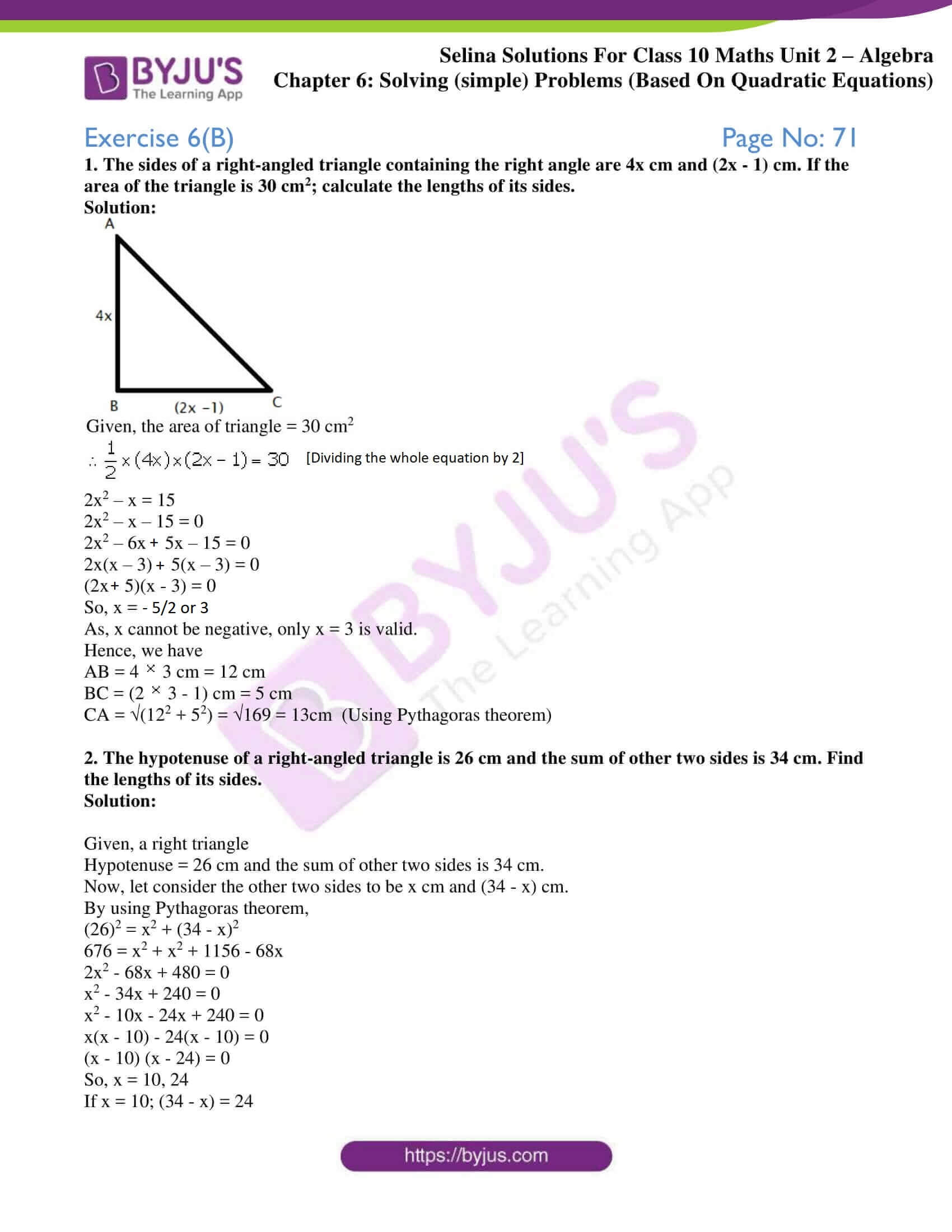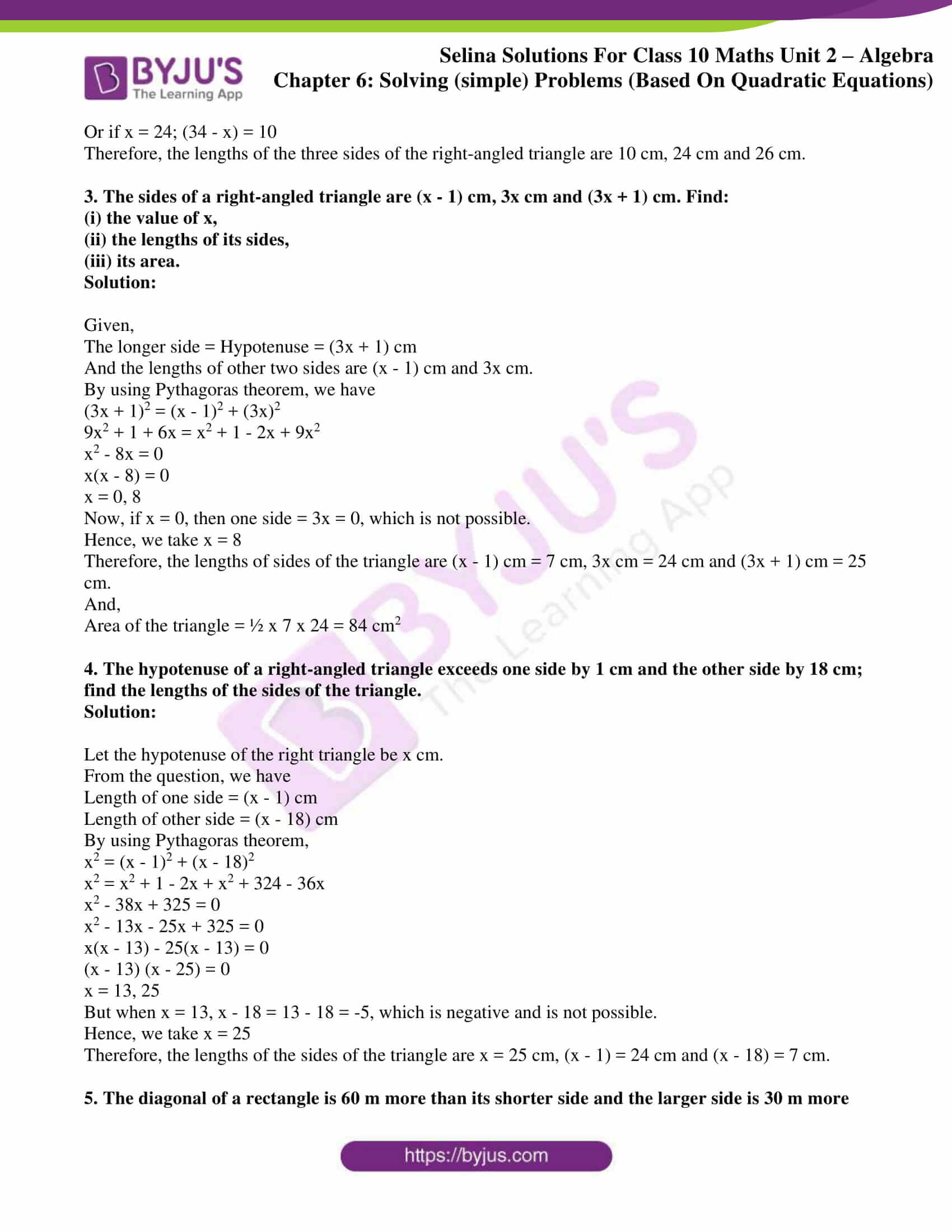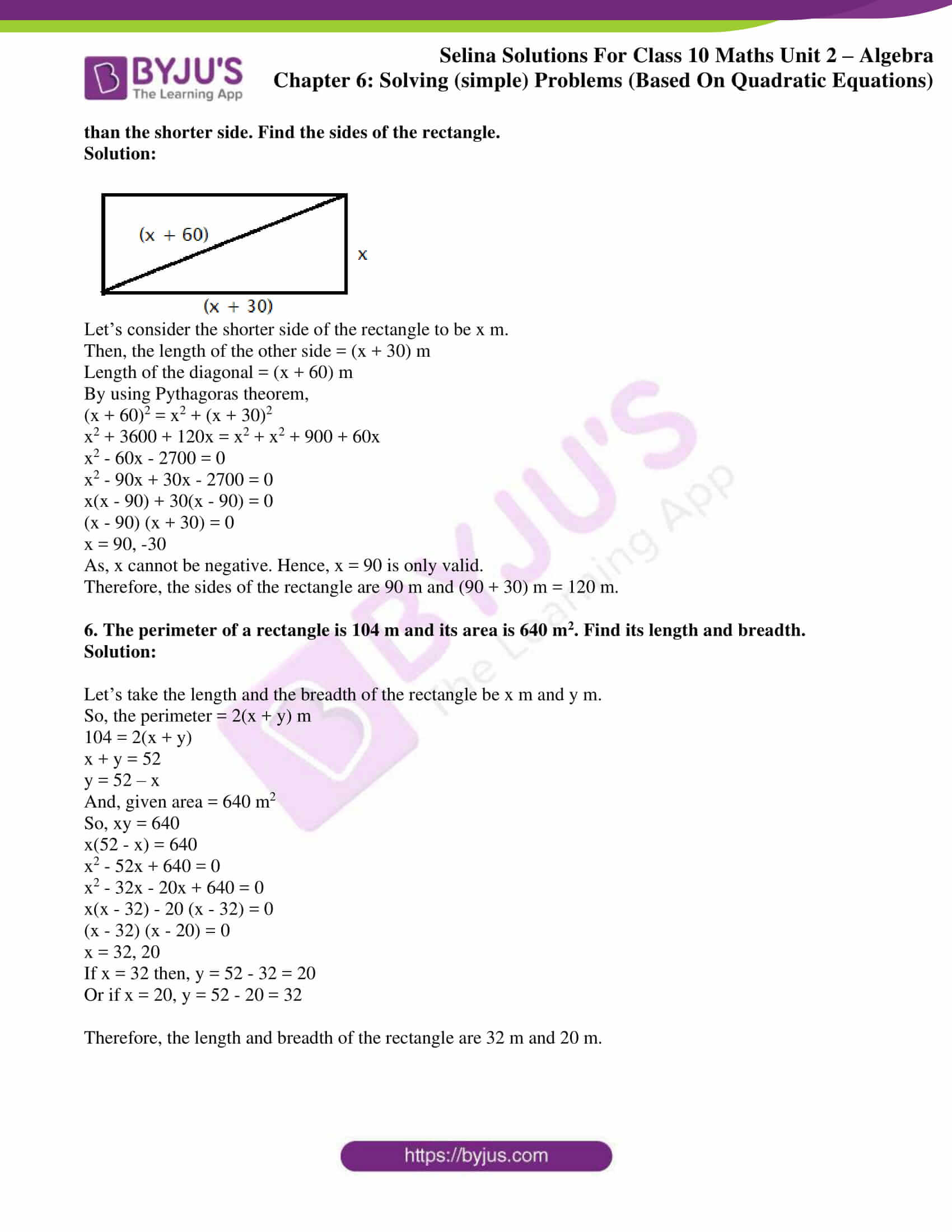### Access other exercises of Selina Solutions Concise Maths Class 10 Chapter 6 Solving (simple) Problems (Based On Quadratic Equations)

Exercise 6(A) Solutions

Exercise 6(C) Solutions

Exercise 6(D) Solutions

Exercise 6(E) Solutions

### Access Selina Solutions Concise Maths Class 10 Chapter 6 Solving (simple) Problems (Based On Quadratic Equations) Exercise 6(B)

1. The sides of a right-angled triangle containing the right angle are 4x cm and (2x – 1) cm. If the area of the triangle is 30 cm2; calculate the lengths of its sides.

Solution: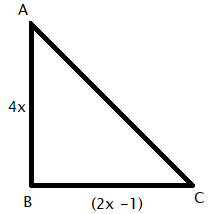Given, the area of triangle = 30 cm2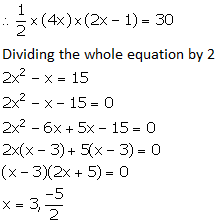As, x cannot be negative, only x = 3 is valid.

Hence, we have

AB = 4 × 3 cm = 12 cm

BC = (2 × 3 – 1) cm = 5 cm

CA = √(122 + 52) = √169 = 13cm  (Using Pythagoras theorem)

2. The hypotenuse of a right-angled triangle is 26 cm and the sum of other two sides is 34 cm. Find the lengths of its sides.

Solution:

Given, a right triangle

Hypotenuse = 26 cm and the sum of other two sides is 34 cm.

Now, let consider the other two sides to be x cm and (34 – x) cm.

By using Pythagoras theorem,

(26)2 = x2 + (34 – x)2

676 = x2 + x2 + 1156 – 68x

2x2 – 68x + 480 = 0

x2 – 34x + 240 = 0

x2 – 10x – 24x + 240 = 0

x(x – 10) – 24(x – 10) = 0

(x – 10) (x – 24) = 0

So, x = 10, 24

If x = 10; (34 – x) = 24

Or if x = 24; (34 – x) = 10

Therefore, the lengths of the three sides of the right-angled triangle are 10 cm, 24 cm and 26 cm.

3. The sides of a right-angled triangle are (x – 1) cm, 3x cm and (3x + 1) cm. Find:

(i) the value of x,

(ii) the lengths of its sides,

(iii) its area.

Solution:

Given,

The longer side = Hypotenuse = (3x + 1) cm

And the lengths of other two sides are (x – 1) cm and 3x cm.

By using Pythagoras theorem, we have

(3x + 1)2 = (x – 1)2 + (3x)2

9x2 + 1 + 6x = x2 + 1 – 2x + 9x2

x2 – 8x = 0

x(x – 8) = 0

x = 0, 8

Now, if x = 0, then one side = 3x = 0, which is not possible.

Hence, we take x = 8

Therefore, the lengths of sides of the triangle are (x – 1) cm = 7 cm, 3x cm = 24 cm and (3x + 1) cm = 25 cm.

And,

Area of the triangle = ½ x 7 x 24 = 84 cm2

4. The hypotenuse of a right-angled triangle exceeds one side by 1 cm and the other side by 18 cm; find the lengths of the sides of the triangle.

Solution:

Let the hypotenuse of the right triangle be x cm.

From the question, we have

Length of one side = (x – 1) cm

Length of other side = (x – 18) cm

By using Pythagoras theorem,

x2 = (x – 1)2 + (x – 18)2

x2 = x2 + 1 – 2x + x2 + 324 – 36x

x2 – 38x + 325 = 0

x2 – 13x – 25x + 325 = 0

x(x – 13) – 25(x – 13) = 0

(x – 13) (x – 25) = 0

x = 13, 25

But when x = 13, x – 18 = 13 – 18 = -5, which is negative and is not possible.

Hence, we take x = 25

Therefore, the lengths of the sides of the triangle are x = 25 cm, (x – 1) = 24 cm and (x – 18) = 7 cm.

5. The diagonal of a rectangle is 60 m more than its shorter side and the larger side is 30 m more than the shorter side. Find the sides of the rectangle.

Solution: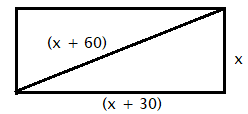Let’s consider the shorter side of the rectangle to be x m.

Then, the length of the other side = (x + 30) m

Length of the diagonal = (x + 60) m

By using Pythagoras theorem,

(x + 60)2 = x2 + (x + 30)2

x2 + 3600 + 120x = x2 + x2 + 900 + 60x

x2 – 60x – 2700 = 0

x2 – 90x + 30x – 2700 = 0

x(x – 90) + 30(x – 90) = 0

(x – 90) (x + 30) = 0

x = 90, -30

As, x cannot be negative. Hence, x = 90 is only valid.

Therefore, the sides of the rectangle are 90 m and (90 + 30) m = 120 m.

6. The perimeter of a rectangle is 104 m and its area is 640 m2. Find its length and breadth.

Solution:

Let’s take the length and the breadth of the rectangle be x m and y m.

So, the perimeter = 2(x + y) m

104 = 2(x + y)

x + y = 52

y = 52 – x

And, given area = 640 m2

So, xy = 640

x(52 – x) = 640

x2 – 52x + 640 = 0

x2 – 32x – 20x + 640 = 0

x(x – 32) – 20 (x – 32) = 0

(x – 32) (x – 20) = 0

x = 32, 20

If x = 32 then, y = 52 – 32 = 20

Or if x = 20, y = 52 – 20 = 32

Therefore, the length and breadth of the rectangle are 32 m and 20 m.